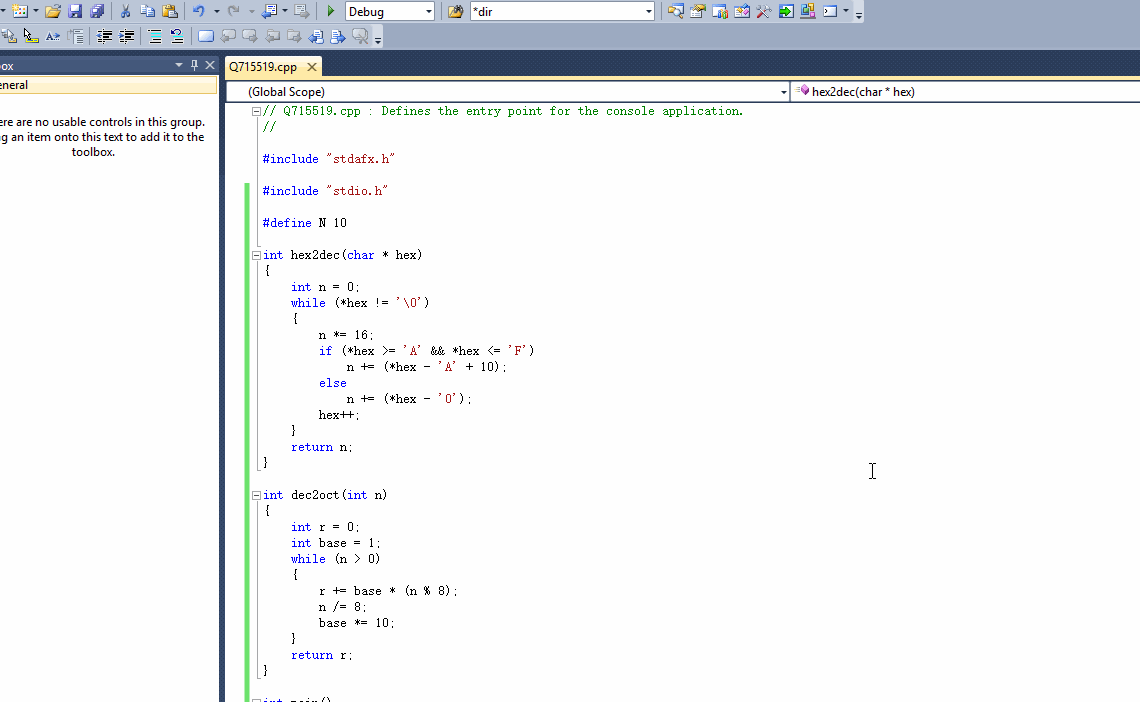给定n个十六进制正整数，输出它们对应的八进制数。

输入的第一行为一个正整数n （1<=n<=10）。
接下来n行，每行一个由0~9、大写字母A~F组成的字符串，表示要转换的十六进制正整数，每个十六进制数长度不超过100000。

输出n行，每行为输入对应的八进制正整数。

【注意】
输入的十六进制数不会有前导0，比如012A。
输出的八进制数也不能有前导0。

2
39
123ABC

71
4435274

【提示】
先将十六进制数转换成某进制数，再由某进制数转换成八进制。

1个回答

``````#include "stdio.h"

#define N 10

int hex2dec(char * hex)
{
int n = 0;
while (*hex != '\0')
{
n *= 16;
if (*hex >= 'A' && *hex <= 'F')
n += (*hex - 'A' + 10);
else
n += (*hex - '0');
hex++;
}
return n;
}

int dec2oct(int n)
{
int r = 0;
int base = 1;
while (n > 0)
{
r += base * (n % 8);
n /= 8;
base *= 10;
}
return r;
}

int main()
{
int n;
int ans[N];
scanf("%d", &n);
for (int i = 0; i < n; i++)
{
char hex;
scanf("%s", hex);
int n = hex2dec(hex);
ans[i] = dec2oct(n);
}
for (int i = 0; i < n; i++)
{
printf("%d\n", ans[i]);
}
return 0;
}
``````12 个月之前 回复WZ℡T 大佬 萌新看不懂 能说一下思路吗？ 谢谢大佬
12 个月之前 回复